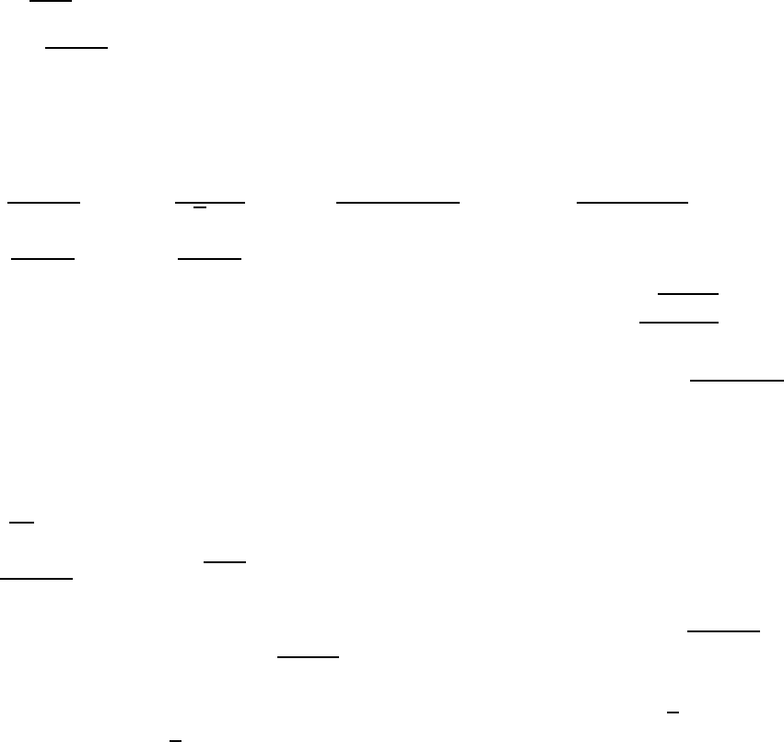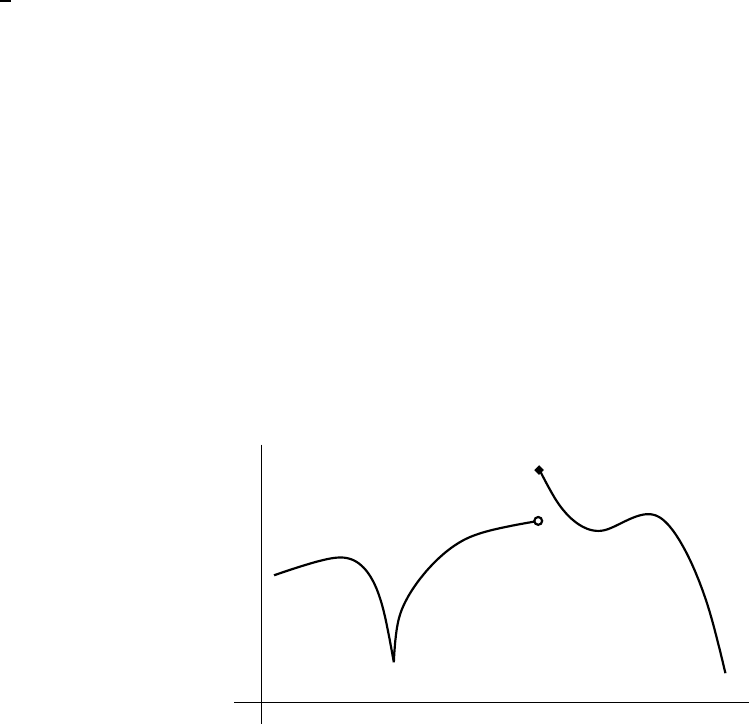Study Guides (380,000)
US (220,000)
Rutgers (3,000)
All (30)

01:640:135 Study Guide - Riemann Sum, Mean Value Theorem, Intermediate Value Theorem

Department
Mathematics
Course Code
01:640:135
Professor
All

This preview shows page 1. to view the full 5 pages of the document.12/6/2005
Some Math 135 review problems
The following problems are based heavily on problems written by Professor Stephen Green-
eld for his Math 135 class in spring 2005. His willingness to let these problems be used
this semester is gratefully acknowledged. The problems are grouped by topic.
Denition of derivative
1. Write the denition of derivative as a limit and use this denition to nd the derivative
of f(x)=x
2
x.
2. Write the denition of derivative as a limit and use this denition to nd the derivative
of f(x)=
1
3x+4
.
3. Write the denition of derivative as a limit and use this denition to nd the derivative
of f(x)=
p
12x.
Computations of limits
1. Evaluate the limits exactly. Give brief evidence supporting your answers which is not
based on a calculator graph or on calculator computations.
a) lim
x!1
2x
2
5
3x
2
+1
b) lim
x!4
x4
p
x2
c) lim
x!5
x
2
3x10
x5
d) lim
x!+1
2x
3
x+3
x
3
+2
e) lim
x!2
x
2
4
j2xj
f) lim
x!2
+
x
2
4
j2xj
2. Find the equations of all vertical and horizontal asymptotes of f(x)=
p
x
2
+4
x3
.
3. Find the equations of all vertical and horizontal asymptotes of f(x) =
3+5e
2x
7e
2x
.
Computations with exp and log should be simplied as much as possible; approximations
are not acceptable.
Computations of derivatives
1. Find
dy
dx
.
a) y=
2x
2
+5
5x
3
+1
b) y=e
p
x
2
1
c) xy
3
= cos(7x+5y) d) xe
y
= sin(xy)
e) y=xln(3x+5) f) 2x
3
+5x
2
y+y
3
=2 g) y= ln(x
4
+3x+1) h) y=
3x
3
1
3x
4
+1
i) y=x
5
tan(3x) j) y=(4x+3)
p
x
3
+7
2. Suppose f(x) is a dierentiable function satisfying f
0
(1) = 3 and f
0
4
=2, If
g(x)=f(tanx), nd g
0
4
.
3. An equation of the tangent line to y=f(x) when x=1isknown to be 2x+y+3=0.
Find f(1) and f
0
(1).
4. Suppose Uis a dierentiable function with U(8) = 5 and U
0
(8) = 3, and that V(x) =
U(x
3
). What are V(2) and V
0
(2)? Use this information to write an equation of the tangent
line to y=V(x) when x=2.
1

Unlock to view full version

Only page 1 are available for preview. Some parts have been intentionally blurred.5. A function is dened implicitly by the equation x
2
y
2
5xy +x+y= 12. Find y
0
in
terms of xand y. Find an equation for the line tangent to the graph of this function at
the point (2;1).
Log/exp etc.
1. Find the range of f(x)=e
2x
+e
3x
.
Continuity & dierentiability
1. Here f(x)=
x+3 if x2
1
2
x
2
+Aif 2<x
where Ais a constant to be determined. Find Aso
that f(x)iscontinuous for all values of x. Sketch a graph of y=f(x) using that value of
Afor 4x2. Is f(x) dierentiable at x=2 using that value of A?
2. Here f(x)=
(
Ax
2
1 if x<1
x+Bif 1x1
2 if 1 <x
where Aand Bare constants to be determined.
Find numbers Aand Bso that f(x) is continuous for all values of x. Sketch a graph of
y=f(x) for 3x3.
3. In this problem f(x) =
8
<
:
1+x
2
if x<2
A+Bx if 2x<1
x
2
if x1
. Find Aand Bso that f(x) is
continuous at all points. Sketch a graph of y=f(x) for 3x3. For which values of
xis f(x)not dierentiable?
4. In the graph of y=f(x) to the right,
identify with many point which is a
relative minimum; Many point which
is a relative maximum; Cany point
which is a critical point; Iany point
which is an inection point; NC any
point at which f(x)isnot continuous;
and ND any point at which f(x)isnot
dierentiable. Some points may have
more than one label.
2